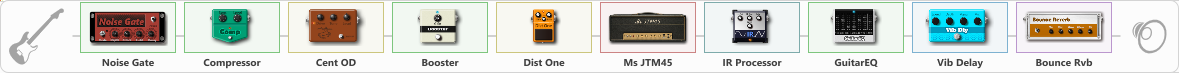Discussion in 'ToneLib-GFX presets' started by Skeve Nelis, Oct 20, 2022.

Preset name: Stadium/Arena Rock SNS I

SkeveNelis

Effects chain:Effect: "Noise Gate" (Dynamics / Filter), active - "yes"
{
"Mode" = Manual
"Depth" = 20
"Threshold" = 74
"Attack" = 0
"Hold" = 5
"Decay" = 250
}

Effect: "Compressor" (Dynamics / Filter), active - "yes"
{
"Sense" = 36
"Level" = 51
}

Effect: "Cent OD" (Overdrive / Distortion), active - "yes"
{
"Drive" = 17
"Tone" = 37
"Level" = 55
}

Effect: "Booster" (Dynamics / Filter), active - "yes"
{
"Gain" = 57
}

Effect: "Dist One" (Overdrive / Distortion), active - "yes"
{
"Drive" = 11
"Tone" = 34
"Level" = 61
}

Effect: "Ms JTM45" (Amp simulators), active - "yes"
{
"Gain" = 64
"Bass" = 43
"Middle" = 91
"Treble" = 90
"Presence" = 56
"Master" = 73
"Level (dB)" = 3
}

Effect: "IR Processor" (Cabinets), active - "yes"
{
"IR" = Marshall JCM2000 SM57
"Low Cut (Hz)" = 205
"Hi Cut (kHz)" = 19.5
"Mix" = 100
"Level (dB)" = 0
}

Effect: "GuitarEQ" (Dynamics / Filter), active - "yes"
{
"160 Hz" = 7
"400 Hz" = -2
"800 Hz" = 4
"1.6 kHz" = 4
"3.2 kHz" = 0
"6.4 kHz" = -5
"12 kHz" = -10
"Level (dB)" = 0
}

Effect: "Vib Delay" (Modulation / Sfx), active - "yes"
{
"Speed" = 2.9
"Depth" = 29
"Feedback" = 24
"Time" = 5.9
"Mix" = 27
"Mode" = 2
}

Effect: "Bounce Rvb" (Reverberation), active - "yes"
{
"Time" = 4.3
"PreDelay" = 0
"LoDamp" = 57
"HiDamp" = 56
"Mix" = 34
}

Note: You will need to download and install the ToneLib-GFX software to use the preset.

File size:
7.1 KB
Views:
2,728